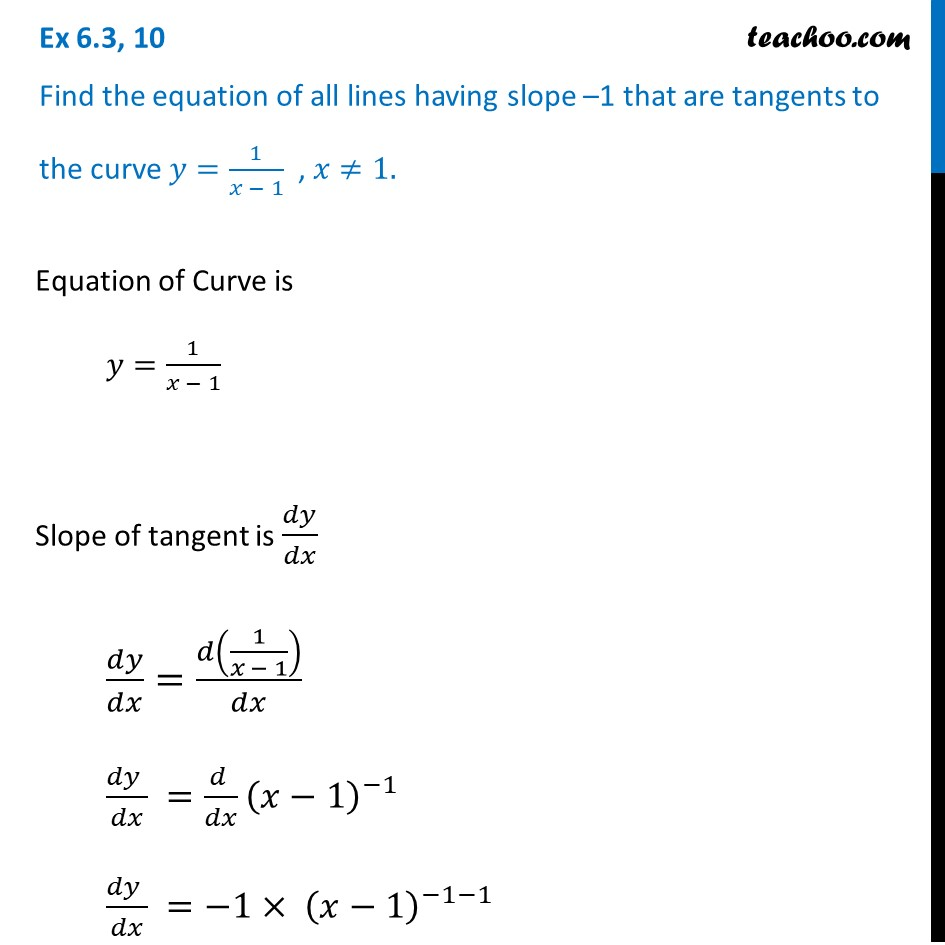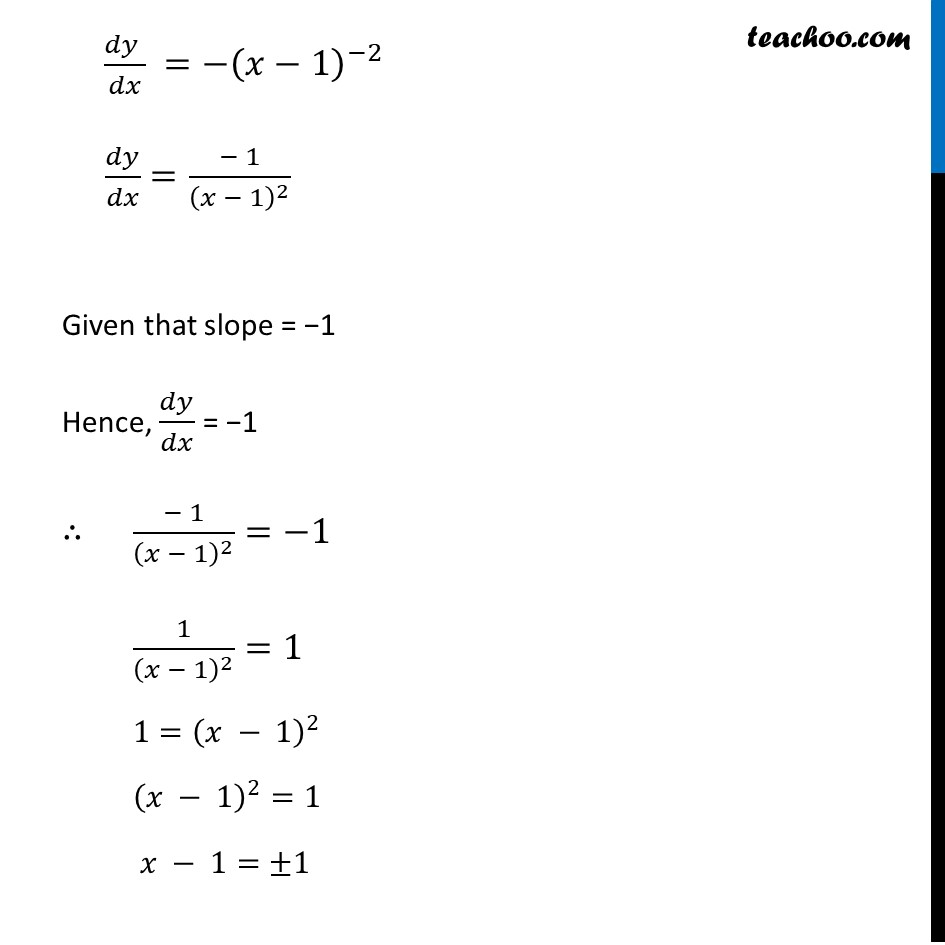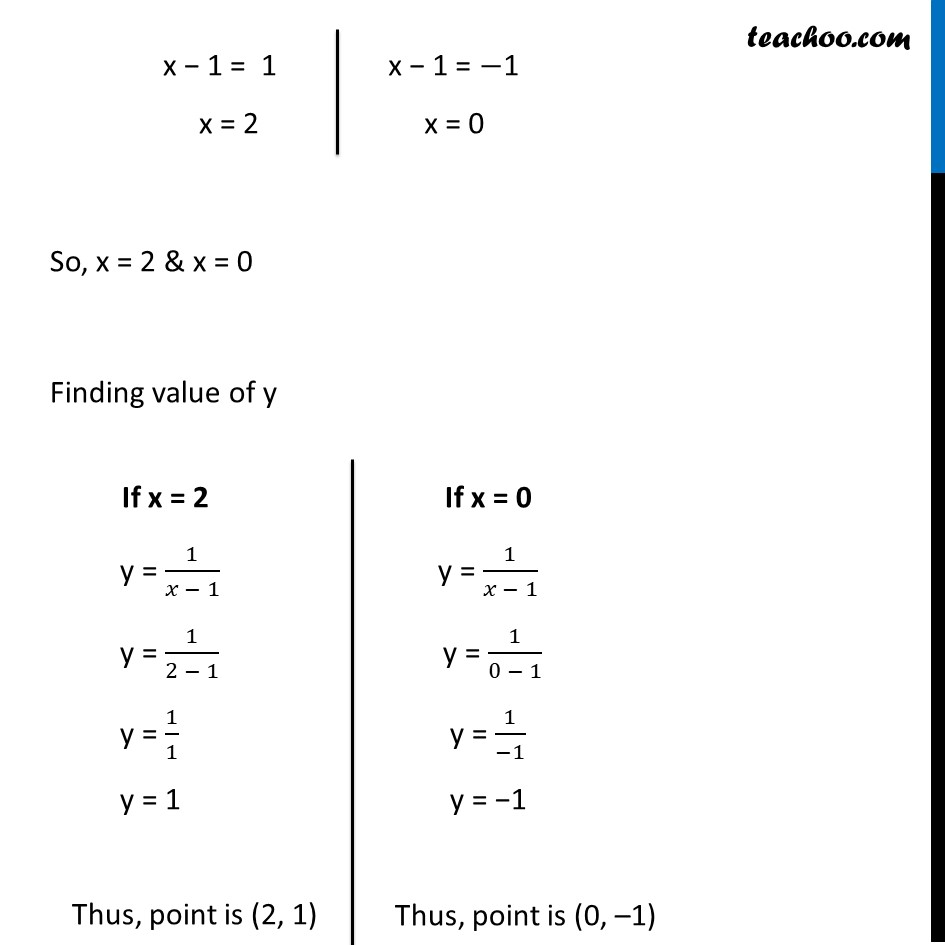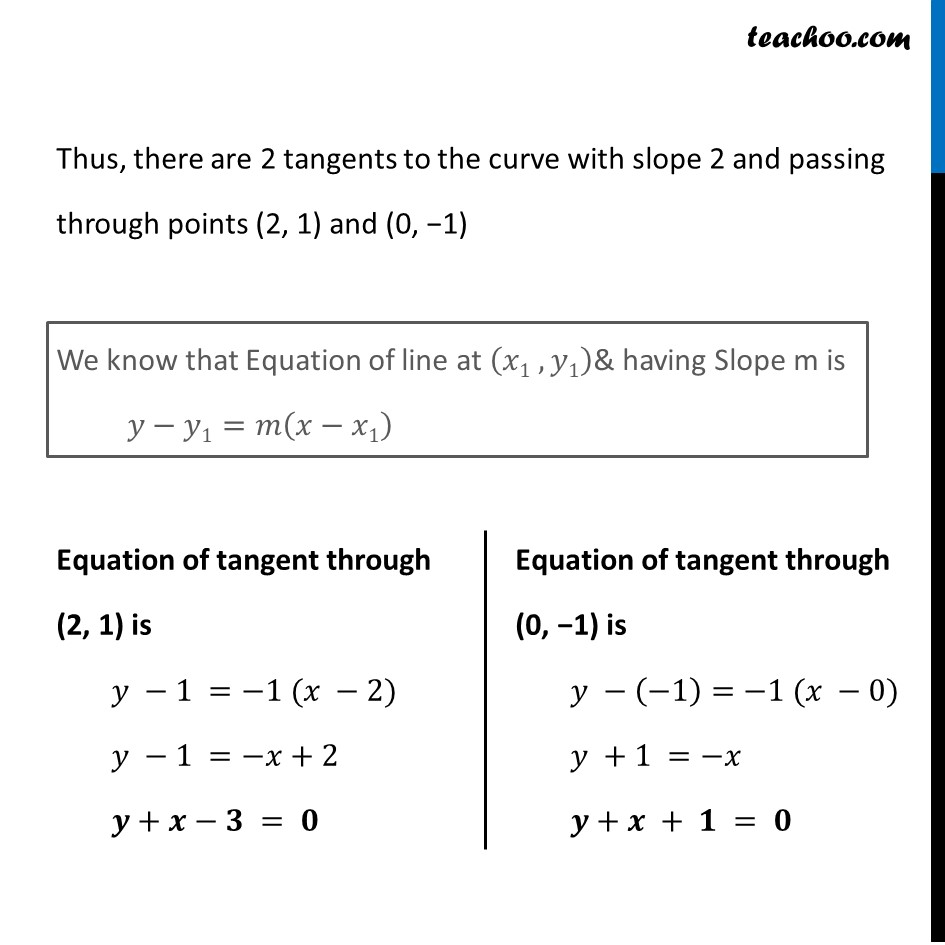Tangents and Normals (using Differentiation)

Chapter 6 Class 12 Application of Derivatives
Serial order wiseLearn in your speed, with individual attention - Teachoo Maths 1-on-1 Class

### Transcript

Question 10 Find the equation of all lines having slope –1 that are tangents to the curve 𝑦=1/(𝑥 − 1) , 𝑥≠1.Equation of Curve is 𝑦=1/(𝑥 − 1) Slope of tangent is 𝑑𝑦/𝑑𝑥 𝑑𝑦/𝑑𝑥=𝑑(1/(𝑥 − 1))/𝑑𝑥 (𝑑𝑦 )/𝑑𝑥 =(𝑑 )/𝑑𝑥 (𝑥−1)^(−1) (𝑑𝑦 )/𝑑𝑥 =−1〖 × (𝑥−1)〗^(−1−1) (𝑑𝑦 )/𝑑𝑥 =−(𝑥−1)^(−2) 𝑑𝑦/𝑑𝑥=(− 1)/(𝑥 − 1)^2 Given that slope = −1 Hence, 𝑑𝑦/𝑑𝑥 = −1 ∴ (− 1)/(𝑥 − 1)^2 =−1 1/(𝑥 − 1)^2 =1 1=(𝑥 − 1)^2 (𝑥 − 1)^2=1 𝑥 − 1=±1 x − 1 = 1 x = 2 x − 1 = −1 x = 0 So, x = 2 & x = 0 Finding value of y If x = 2 y = 1/(𝑥 − 1) y = 1/(2 − 1) y = 1/1 y = 1 Thus, point is (2, 1) If x = 0 y = 1/(𝑥 − 1) y = 1/(0 − 1) y = 1/(−1) y = −1 Thus, point is (0, –1) Thus, there are 2 tangents to the curve with slope 2 and passing through points (2, 1) and (0, −1) We know that Equation of line at (𝑥1 , 𝑦1)& having Slope m is 𝑦−𝑦1=𝑚(𝑥−𝑥1) Equation of tangent through (2, 1) is 𝑦 −1 =−1 (𝑥 −2) 𝑦 −1 =−𝑥+2 𝒚+𝒙−𝟑 = 𝟎 Equation of tangent through (0, −1) is 𝑦 −(−1)=−1 (𝑥 −0) 𝑦 +1 =−𝑥 𝒚+𝒙 + 𝟏 = 𝟎